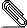# Re: [openssl-project] Entropy seeding the DRBG

```The internal state of the CTR-DRBG consists of a key K and a counter V (see
figure 11 on page 48, which is the page before table 3). This is the reason why
it requires bits_of(K) + bits_of(V) = keysize + blocksize = 256 + 128 = 384
bits to initialize the internal state of the DRBG. ```
```
But the seedlen (in bits) *does not* in general coincide with the
security_strength! This is only in the case when _no_ derivation function is
used! See the aforementioned table 3 on page 49:

If a derivation function is not used:
Minimum entropy input length:           seedlen
(min _length = blocklen + keylen)

But we are in the case when a derivation function is used. Here only
'security_strength' (= 256) bits of entropy are required.

If a derivation function is used:
Minimum entropy input length:           security_strength
(min _length)

These bits are 'stretched out' to seed_length (=384) bits by the "derivation
function", which is essentially an aes-ctr bit stream. Or, to be more precise:
if we provide a large buffer of low entropy input, then this low entropy input
will be condensed, stirred, and spread out equally over the 384 bits of the
initial state by the derivation function.

Matthias

> -----Ursprüngliche Nachricht-----
> Von: openssl-project <openssl-project-boun...@openssl.org> Im Auftrag von
> Richard Levitte
> Gesendet: Mittwoch, 4. April 2018 15:09
> An: openssl-project@openssl.org
> Betreff: Re: [openssl-project] Entropy seeding the DRBG
>
> 2018 11:58:54 +0000, "Salz, Rich"
> <rs...@akamai.com> said:
>
> rsalz> Is it expected that the number of bits of seed must equal the
> rsalz> number of bits in the key strength?
>
> It is expected that the number of bits of entropy in the seed (the VMS
> function declares 4 bits of entropy per byte, considering the sources
> it uses) equals a requirement, and it seems that the requirement is to
> have the DRBG strength (which is measure in number of entropy bits)
> match the number of bits of the block cipher used to generate the
> randomness bits.  If I understand things correctly...  and that does
> seem to match what's specified in SP800-90A r1.  I suggest a quick
> study of table 3 in section 10 (Definitions for the CTR_DRBG), seen on
> page 58 in
> https://nvlpubs.nist.gov/nistpubs/specialpublications/nist.sp.800-90ar1.pdf
> Very specifically, there's the row with the title "Seed length
> (seedlen = outlen + keylen)" that very clearly says 384 bits for
> AES-256.
>
> "Seed length" itself is defined in section 8:
>
>     8.6.4 Seed Length
>
>     The minimum length of the seed depends on the DRBG mechanism and the
>     security strength required by the consuming application, but shall be
>     at least the number of bits of entropy required. See the tables in
>     Section 10.
>
> rsalz> But at any rate, raising the seed size to 256 seems mildly
> rsalz> tolerable, although I would prefer to keep it at 128.  Raising
> rsalz> it to 384 is wrong.
>
> Note that with a nonce, that'll be 192 bits, unless I'm thinking
> wrong...  But I agree with you, at least from a very practical point
> of view.
>
> --
> Richard Levitte         levi...@openssl.org
> OpenSSL Project         http://www.openssl.org/~levitte/
> _______________________________________________
> openssl-project mailing list
> openssl-project@openssl.org
> https://mta.openssl.org/mailman/listinfo/openssl-project
```smime.p7s
Description: S/MIME cryptographic signature

```_______________________________________________
openssl-project mailing list
openssl-project@openssl.org
https://mta.openssl.org/mailman/listinfo/openssl-project```